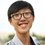# Chemistry - Ideal GasesFor Ideal Gases, the important assumptions are as below:

1. The intermolecular forces (Attractive Force or Repulsive Force) between the gas molecules are entirely negligible.

2. The volume occupied by the gas molecules themselves is entirely negligible relative to the volume of the container.

By the assumptions above, we can sure that there are no "Ideal Gas" in our real world.

However, scientists formulate the concept of Ideal Gases to make sure some gas law will always true. Or else, more errors will present in our calculations or data.

On the other hand, errors do exist when we dealt with Real Gases. We shall discuss this later.

If you are well with Ideal Gases, then here is the Ideal Gas Law:

$PV=nRT$

$P$ is for Pressure, with the unit $Pa$.

$V$ is for Volume, with the unit $m^3$.

$n$ is the Number of moles, with the unit $mol$.

$R$ is a Constant, which is $8.314 m^3mol^{-1}K^{-1}$.

$T$ is for Temperature, with the unit $K$.

Now, there is an example:

At a standard status (we mean by $P=1 \text{atm}$ and $T=0^\circ C$), what is the volume of a $25g$ $O_2$?

First, we convert the units.

$P=1 \text{atm}=101325 Pa$

$T=0^\circ C=(0+273)K=273K$.

We now have all the details we wanted, by the formula we have

$PV=nRT$

$(101325)V=\frac{25}{32}\times8.314\times273$

$V=0.0175m^3$

Thus, the volume is $0.0175m^3$.Note by Christopher Boo
6 years, 11 months ago

This discussion board is a place to discuss our Daily Challenges and the math and science related to those challenges. Explanations are more than just a solution — they should explain the steps and thinking strategies that you used to obtain the solution. Comments should further the discussion of math and science.

When posting on Brilliant:

• Use the emojis to react to an explanation, whether you're congratulating a job well done , or just really confused .
• Ask specific questions about the challenge or the steps in somebody's explanation. Well-posed questions can add a lot to the discussion, but posting "I don't understand!" doesn't help anyone.
• Try to contribute something new to the discussion, whether it is an extension, generalization or other idea related to the challenge.

MarkdownAppears as
*italics* or _italics_ italics
**bold** or __bold__ bold
- bulleted- list
• bulleted
• list
1. numbered2. list
1. numbered
2. list
Note: you must add a full line of space before and after lists for them to show up correctly
paragraph 1paragraph 2

paragraph 1

paragraph 2

[example link](https://brilliant.org)example link
> This is a quote
This is a quote
    # I indented these lines
# 4 spaces, and now they show
# up as a code block.

print "hello world"
# I indented these lines
# 4 spaces, and now they show
# up as a code block.

print "hello world"
MathAppears as
Remember to wrap math in $$ ... $$ or $ ... $ to ensure proper formatting.
2 \times 3 $2 \times 3$
2^{34} $2^{34}$
a_{i-1} $a_{i-1}$
\frac{2}{3} $\frac{2}{3}$
\sqrt{2} $\sqrt{2}$
\sum_{i=1}^3 $\sum_{i=1}^3$
\sin \theta $\sin \theta$
\boxed{123} $\boxed{123}$

Sort by:

Can I know what is this topic?

- 6 years, 11 months ago

This is quite of a common sense once you get the intuition for it. ^^ But this formula, is very useful in physics and chemistry. (y) A chemistry toolkit that is used a lot in thermodynamics. ^^ I guess, that's a bit on the application side. Hahhaa. =)

- 6 years, 11 months ago

I've noticed I missed our many thing too. I will try my best to edit it. :)

- 6 years, 11 months ago

Hi Welthan, this topic is about about the laws governing ideal gases as defined by Christopher. It helps us to link up the temperature, volume and pressure of the gases along with the mass i.e. the number of moles. It is derived from the various gas laws given by Boyle, Charles, Gay-Lussac, etc. It has its applications in various fields.

- 6 years, 11 months ago

It has been a long time since my last post. This is because I went to my hometown to celebrate Chinese New Year. However, Anish and Vishnuram had post some chemistry notes on Electrochemistry and Organic Chemistry. Check it out here!

- 6 years, 11 months ago

Happy Chinese New Year!! ^^

- 6 years, 11 months ago

Superb. Waiting for gas law problems.

- 6 years, 11 months ago

This is good stuff Christopher. Keep it up!

Staff - 6 years, 11 months ago